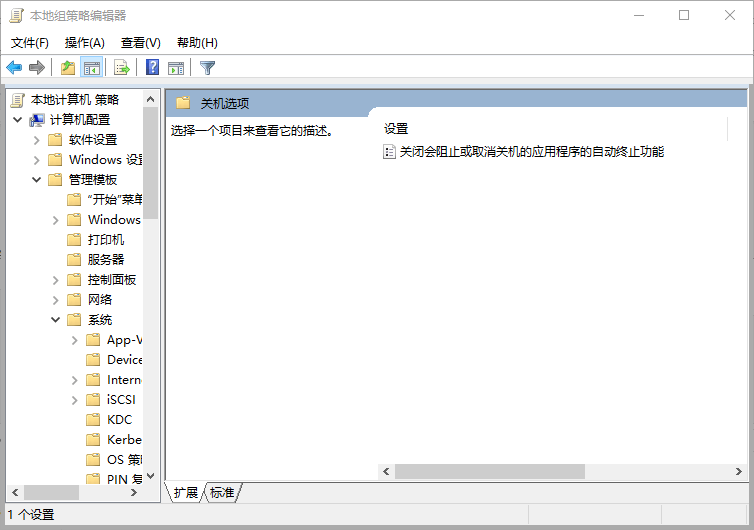## 一球从100米高度自由落下，每次落地后反跳回原高度的一半，再落下。编写程序，输出第n次落地时，小球落下弹起共经过多少米。

```<?php
function rebouns(\$n) {
\$begin = 100;
for(\$i=1; \$i<=\$n; \$i++){
if(\$i==1 || \$i==2){
}else{
}
echo "第".\$i."次落地，经过距离为".\$sum."<br />";
}
}
rebouns(5);```

```第1次落地，经过距离为100

## Please compose a functio thar meets the following requirement

We are a php shop and prefer you answering in php,

But you might answer in any other languages in case you are not familiarwith it, we can read C#, Java, C++, Perl, Ruby, etc.

Requirement:

The input would be an English sentence as a string, please transforms it as described below and return a new…

The sentence would contains only alphabet(a-z and A-Z) and space, each word would be separated by exactly…

space. There would be no spaces before and after the sentence.

Please return the string with each word spelled in reverse, however, the position of the capitalization of each …

should stay the same for each word.

For example:

Input: This is an Apple on eBay

Output: sihT si na elppA no yaBe

```<?php
function rev(\$arr) {
\$arr = explode(" ",\$arr);
foreach(\$arr as &\$v){
\$v = strrev(\$v);
}
return implode(" ",\$arr);
}
\$arr = "This is an Apple on eBay";
\$res = rev(\$arr);
print_r(\$res);```

## 用 PHP 语言解决鸡兔同笼问题

```<?php
function fn(\$h,\$y) {
\$maxC = \$h;
\$minC = ceil(\$y/4);
\$c = \$minC;
\$r = false;
for(; \$c<\$maxC; \$c++)
{
\$r = \$h - \$c;
if((\$c*2 + \$r*4) == \$y) return \$c;
}
return false;
}
\$chick = fn(10,26);
if(false == \$chick){
echo "输入有误";
}else{
\$rabbit = 10 - \$chick;
echo "鸡:{\$chick},兔:{\$rabbit}";
}```

## 使用 PHP 描述选择排序算法

```<?php
function select(&\$arr)
{
for(\$i=0; \$i<count(\$arr)-1; ++\$i)
{
//假设下标为\$i的元素就是最小的数
\$min = \$arr[\$i];
\$minIndex = \$i;
//取得最小的元素及其下标
for(\$j=\$i+1; \$j<count(\$arr); ++\$j)
{
if(\$min>\$arr[\$j])
{
\$min = \$arr[\$j];
\$minIndex = \$j;
}
}
// 最后交换
\$temp = \$arr[\$i];
\$arr[\$i] = \$arr[\$minIndex];
\$arr[\$minIndex] = \$temp;
}
return \$arr;
};
\$arr = array(12,5,33,78,96,16,8,57,62);
\$res = select(\$arr);
print_r(\$res);```

## 用 PHP 实现斐波那契数列

```<?php
\$arr = 0;
\$arr = 1;
for(\$i = 2; \$i<100; \$i++ ){
\$arr[\$i] = \$arr[\$i-1] + \$arr[\$i-2];
}
echo join(",",\$arr);```

## PHP读取大文件末尾N行的高效方法

```while(!feof(\$fp)){
\$data.=fgets(\$fp,4096);
}```

```/**
* 取文件最后\$n行
* @param string \$filename 文件路径
* @param int \$n 最后几行
* @return mixed false表示有错误，成功则返回字符串
*/
function FileLastLines(\$filename,\$n){
if(!\$fp=fopen(\$filename,'r')){
echo "打开文件失败，请检查文件路径是否正确，路径和文件名不要包含中文";
return false;
}
\$pos=-2;
\$eof="";
\$str="";
while(\$n>0){
while(\$eof!="\n"){
if(!fseek(\$fp,\$pos,SEEK_END)){
\$eof=fgetc(\$fp);
\$pos--;
}else{
break;
}
}
\$str.=fgets(\$fp);
\$eof="";
\$n--;
}
return \$str;
}
echo nl2br(FileLastLines('sss.txt',4));```

via: http://www.thinkphp.cn/topic/4127.html

## 约瑟夫环

```<?php
//\$n 人数 \$k 开始位置  \$m 要数得数字
function array_f(\$n,\$k,\$m){
for(\$i=1; \$i <= \$n ; \$i++){
\$oldArr[] = \$i;
}
for (\$i=0;\$i<\$k-1;\$i++){
\$num = array_shift(\$oldArr);
\$oldArr[] = \$num;
}
for(\$i=0;\$i<\$n;\$i++){
for(\$j=0;\$j<\$m-1;\$j++){
\$num = array_shift(\$oldArr);
\$oldArr[] = \$num;
}
\$newArr[] = array_shift(\$oldArr);
}
var_dump(\$newArr);
}
array_f(6,2,3);```

## 关闭 WIN10 关机时提醒 “仍要关机”

“计算机配置”→“管理模板”→“系统”→“关机选项”

“关闭会阻止或取消关机的应用程序的自动终止功能”在属性中设置“已禁用”## 使用 PHP 实现冒泡排序，将数组 \$a=array() 安装从小到大的方式进行排序

```<?php
\$arr = [1,43,6,7,3,5,78];
function bubbleSort (\$arr) {
\$num = count(\$arr);
\$temp;
for (\$i=0; \$i<\$num-1; \$i++){
for (\$j=0; \$j<\$num-1-\$i; \$j++){
if (\$arr[\$j] > \$arr[\$j+1]){
\$temp = \$arr[\$j];
\$arr[\$j] = \$arr[\$j+1];
\$arr[\$j+1] = \$temp;
}
}
}
return \$arr;
}
print_r (bubbleSort(\$arr));
```